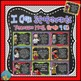# I Can Statements for Tennessee and Common Core Math Grade 4 Q3Subject
Resource Type
File Type
PDF (28 MB|34 pages)
Standards
\$3.50
• Product Description
• Standards
Student-friendly versions of the state standards can be seen and read across the room, as demanded by most school administrators. Use these posters to achieve your goal of improving your students’ knowledge of their essential learning. Each statement is accompanied by a fun illustration. These thirty-four posters are designed for the third quarter of fourth grade math in the state of Tennessee, and are aligned with Common Core.

“I Can” posters in this pack address the following Tennessee standards:

4.MD.1, 4.MD.2, 4.MD.3, 4.MD.4, 4.MD.5, 4.MD.6, and 4.MD.7 (Complex standards are broken down into multiple “I Can” statements.)

Buy the bundle and save! This set is included in the following bundle:

I Can Statements for Tennessee Math Grade 4 All Year Bundled for Savings!

You might also like these additional subject area resources for Tennessee and Common Core standards instruction:

I Can Statements for Tennessee and Common Core

Please do not post this product to the Internet (even to a class web page) as this allows others to take credit for and copy my work. Thank you!
Recognize angle measure as additive. When an angle is decomposed into non-overlapping parts, the angle measure of the whole is the sum of the angle measures of the parts. Solve addition and subtraction problems to find unknown angles on a diagram in real world and mathematical problems, e.g., by using an equation with a symbol for the unknown angle measure.
Measure angles in whole-number degrees using a protractor. Sketch angles of specified measure.
Recognize angles as geometric shapes that are formed wherever two rays share a common endpoint, and understand concepts of angle measurement:
Make a line plot to display a data set of measurements in fractions of a unit (1/2, 1/4, 1/8). Solve problems involving addition and subtraction of fractions by using information presented in line plots. For example, from a line plot find and interpret the difference in length between the longest and shortest specimens in an insect collection.
Apply the area and perimeter formulas for rectangles in real world and mathematical problems. For example, find the width of a rectangular room given the area of the flooring and the length, by viewing the area formula as a multiplication equation with an unknown factor.
Total Pages
34 pages